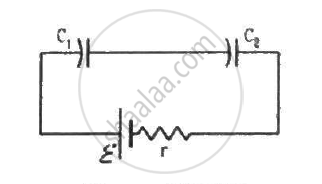Advertisement Remove all ads

# Consider the Situation Shown in Figure. the Switch is Closed at T = 0 When the Capacitors Are Uncharged. Find the Charge on the Capacitor C1 As a Function of Time T. - Physics

Sum

Consider the situation shown in figure. The switch is closed at t = 0 when the capacitors are uncharged. Find the charge on the capacitor C1 as a function of time t.Advertisement Remove all ads

#### Solution

The two capacitors are connected in series. Their equivalent capacitance,

$C_{eqv} = \frac{C_1 C_2}{C_1 + C_2}$

The growth of charge in the capacitors,

$q = C_{eqv} \epsilon\left( 1 - e^{- \frac{1}{r C_{eqv}}} \right)$

Concept: Energy Stored in a Capacitor
Is there an error in this question or solution?
Advertisement Remove all ads

#### APPEARS IN

HC Verma Class 11, Class 12 Concepts of Physics Vol. 2
Chapter 10 Electric Current in Conductors
Q 82 | Page 203
Advertisement Remove all ads

#### Video TutorialsVIEW ALL 

Advertisement Remove all ads
Share
Notifications

View all notifications

Forgot password?